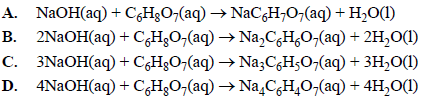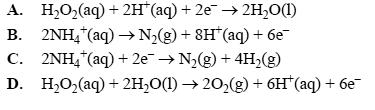General (2015 VCE) 1) When concentrated sulfuric acid is added to dry sucrose, C12H22O111, a black residue of pure carbon is produced. An equation for the reaction is 2C12H22O11(s) + 2H2SO4(aq) + O2(g) => 22C(s) + 2CO2(g) + 24H2O(g) + 2SO2(g) M(C12H22O11) = 342.0 g mol–1 The mass of carbon residue that could be produced by the reaction of 50.0 g of sucrose with excess concentrated sulfuric acid is A. 0.159 g B. 0.877 g C. 19.3 g D. 38.6 g Solution2) In an experiment, 0.051 mol of sodium hydroxide, NaOH, reacted completely with 0.017 mol of citric acid, C6H8O7. Which one of the following equations correctly represents the reaction between citric acid and the sodium hydroxide solution?Solution3) The emergency oxygen system in a passenger aircraft uses the decomposition of sodium chlorate to produce oxygen. At 76.0 kPa and 292 K, each adult passenger needs about 1.60 L of oxygen per minute. The equation for the reaction is 2NaClO3(s) => 2NaCl(s) + 3O2(g) M(NaClO3) =106.5 g mol–1 The mass of sodium chlorate required to provide the required volume of oxygen for each adult passenger per minute is: A. 3.56 g B. 5.34 g C. 7.85 g D. 53.7 g Solution4) In which one of the following compounds is sulfur in its lowest oxidation state? A. SO3 B. HSO4- C. SO2 D. Al2S3 Solution5) What is the pH of a 0.0500 M solution of barium hydroxide, Ba(OH)2? A. 1.00 B. 1.30 C. 12.7 D. 13.0 Solution6) The reaction between hydrogen peroxide and ammonium ions is represented by the following equation. 3H2O2(aq) + 2NH4 +(aq) => N2(g) + 2H+(aq) + 6H2O(l) Which one of the following is the correct half-equation for the reduction reaction?Solution